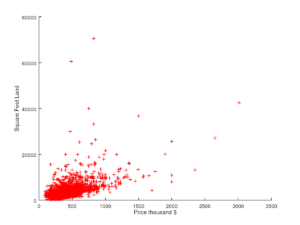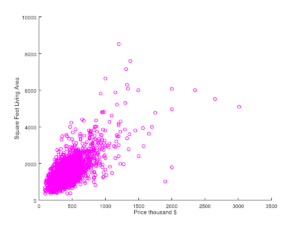## Implementing Linear Regression using Ruby

In this posts I will show how to implement linear regression in Ruby. Using existing Ruby gems we will setup a linear regression model, train the algorithm and make predictions in minutes. For this example we will use historic house prices in Staten Island to predict the value of houses.

You can find the code used in this post and the dataset in the following github repository.

## Obtaining the data

As mentioned above will be implement a machine learning algorithm to predict house prices on Staten Island based on historic data. To obtain the historic data we will use the NYC Open Data portal. New York City has a wonderful program that makes city data freely available for the public. We will base our implementation on this data.

Specifically we are using the Staten Island part of the Annualized Rolling Sales Update dataset. I have removed the worst outliers from the dataset and reordered the data into a CSV file that looks something like this:

```
LAND SQUARE FEET,GROSS SQUARE FEET,SALE PRICE,BOROUGH,NEIGHBORHOOD,TAX CLASS AT PRESENT,BLOCK,LOT,EASE-MENT,BUILDING CLASS AT PRESENT,ZIP CODE,YEAR BUILT,TAX CLASS AT TIME OF SALE,BUILDING CLASS AT TIME OF SALE,SALE DATE

......

```

The 3 first columns are the most interesting to us, these columns are: land square feet, living area square feet and sale price.To better understand the relationship between land area, living area and price I’ve create two plots showing living area vs. price and land area vs. price.

As we can see in the plots it looks like the living area and land area are related to the price in a linear fashion. This means we can use land and living area as our independent variables to predict the dependent variable the sale price using linear regression.

## Installing prerequisites

In Ruby we don’t have to implement the linear regression algorithm from scratch. Instead we use an existing gem that implements the Linear Regression algorithm.

For this example we use the gem called ruby_linear_regression. This gem will implement linear regression using Ruby’s Matrix implementation and the normal equation which allows you to train the algorithm pretty fast.

To install this gem run the following in your command line:

`gem install ruby_linear_regression`

With the gem installed lets create a ruby file and start our implementation.

## Implementing linear regression

First we need to require the ruby libraries we are going to use to implement our solution. For now require csv for loading data and ruby_linear_regression for the regression algorithm.

```
require 'csv'
require 'ruby_linear_regression'

```

Next we need to load our historic data into two arrays. This is the data we are going to use to train our algorithm and is also called the training data. One array for the independent variables X (the variables used to make a prediction based on) and one array for the dependent variable y (the variable we are trying to predict).

We use the CSV library to load the data into the two arrays as follows:

```x_data = []
y_data = []
# Load data from CSV file into two arrays - one for independent variables X and one for the dependent variable Y
# Each row contains square feet for property and living area like this:
# [ SQ FEET PROPERTY, SQ FEET HOUSE ]
CSV.foreach("./data/staten-island-single-family-home-sales-2015.csv", :headers => true) do |row|
x_data.push( [row.to_i, row.to_i] )
y_data.push( row.to_i )
end

```

Next we initialize an instance of the linear regression algorithm and load our training data.

```
# Create regression model
linear_regression = RubyLinearRegression.new

```

At this point our data is loaded into the algorithm the next step is training the algorithm to such that we can use it to make predictions. This is can be done by simply running train_normal_equation like this:

```
# Train the model using the normal equation
linear_regression.train_normal_equation

```

With the machine learning algorithm trained to our data we can now use it to make predictions. To make a prediction we need to create an array of the values we want to base the predictions on and call the predict method with these values. This can be done like this:

```
# Predict the price of a 2000 sq feet property with a 1500 sq feet house
prediction_data = [2000, 1500]
predicted_price = linear_regression.predict(prediction_data)
puts "Predicted selling price for a 1500 sq feet house on a 2000 sq feet property: #{predicted_price.round}\$"

```

At this point we can run the program like this:

```\$ ruby example.rb
Predicted selling price for a 1500 sq feet house on a 2000 sq feet property: 395853\$```

You can find the full source code and data file for this solution here.

•Pavitra says:
•Julien says: